# JEE Main Mathematics Mock - 6

## 25 Questions MCQ Test Mock Test Series for JEE Main & Advanced 2022 | JEE Main Mathematics Mock - 6

Description
Attempt JEE Main Mathematics Mock - 6 | 25 questions in 60 minutes | Mock test for JEE preparation | Free important questions MCQ to study Mock Test Series for JEE Main & Advanced 2022 for JEE Exam | Download free PDF with solutions
QUESTION: 1

Solution:
QUESTION: 2

Solution:
QUESTION: 3

### The general solution of the equation x2(dy/dx)=2 is

Solution:
QUESTION: 4

The area of the region bounded the curve y = 2x - x2 and the line y = x is

Solution:
QUESTION: 5

If line y=2x is a chord of the circle x2+y2-10x=0, the equation of the circle drawn on the chord assuming it as a diameter is

Solution:
QUESTION: 6

If (cos θ + i sin θ)(cos 2θ + i sin 2θ).....(cos mθ + i + sin mθ) = 1 then the value of θ ,is (m is an integer)

Solution: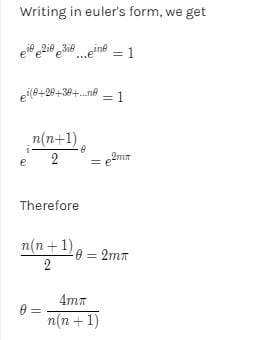QUESTION: 7

If circles x2+y2+2ax+c=0 and x2+y2+2by+c=0 touch each other, then

Solution:
QUESTION: 8

If the amplitude of z − 2 − 3i is π/4 , then the locus of z = x + iy is

Solution:
QUESTION: 9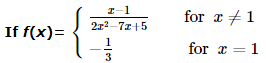then f ′ (1)  =

Solution:
QUESTION: 10

The negation of the statement ' he is rich and happy' is given by

Solution:
QUESTION: 11

If N N + denotes the set of all positive integers and if f : NN + → N is defined by f (n) = the sum of positive divisors of n then f (2 k . 3), where k is a positive integer is

Solution:

f(2k. 3) = The sum of positive divisors of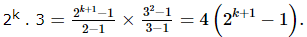QUESTION: 12

In the following question, a Statement of Assertion (A) is given followed by a corresponding Reason (R) just below it. Read the Statements carefully and mark the correct answer-
Assertion(A): The function f(x)=sin x is symmetric about the line x = 0
Reason (R): Every even function is symmetric about y-axis

Solution:
QUESTION: 13
In the following question, a Statement of Assertion (A) is given followed by a corresponding Reason (R) just below it. Read the Statements carefully and mark the correct answer-
Assertion (A): If n is a positive integer then 32n + 7 is divisible by 8
Reason (R): G.C.F. of 16 and 88 is 8
Solution:
QUESTION: 14

If 3, -2, are the eigen values of a non-singular matrix A and |A| = 4, then the eigen values of adj A are

Solution:
QUESTION: 15

The combined equation to the tangents to the parabola y2 = 4ax from an external point A (x1, y1) is

Solution:
QUESTION: 16

In throwing of two dice, the probability of getting a multiple of 4 is

Solution:
QUESTION: 17

If nPr=840, nCr=35, then n=

Solution: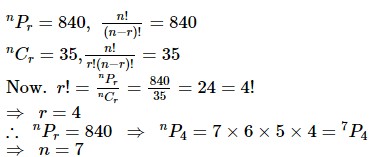QUESTION: 18

Mode is approximately given by

Solution:
QUESTION: 19

The equation of line passing through intersection point of lines x+5y+7=0 and 3x+2y-5=0 and perpendicular to line 7x+2y-5=0 is

Solution:
QUESTION: 20
The subnormal to the curve xy = c2 at any point varies directly as
Solution:
*Answer can only contain numeric values
QUESTION: 21

The number of solutions of the equation tan2x –sec6x + 1 = 0 in [0, 4π] is :-

Solution:

sec6x = 1 + tan2x ⇒ sec6x = sec2x
if |secx| > 1 then sec6x > sec2x
Hence only possible solution is sec2x = 1 ⇒ x = nπ
Hence in [0, 4π] possible solutions are 0, π, 2π, 3π, 4π

*Answer can only contain numeric values
QUESTION: 22

If solution of equation 3cos2θ – 2√3 sin θ cos θ – 3sin2θ = 0 are nπ + π/r and nπ + π/s then |r – s| is equal to:-

Solution:

Equation can be written as
3tan2θ + 2√3 tanθ – 3 = 0
⇒ tan θ = 1/√3 and tan θ = -√3
⇒ θ = nπ + π/6 or θ = nπ – π/3
⇒ |r – s| = |6 + 3| = 9

*Answer can only contain numeric values
QUESTION: 23

If (x1 – x2)2 + (y1 – y2)2 = a2 ;
(x2 – x3)2 + (y2 – y3)2 = b2 ;
(x3 – x1)2 + (y3 – y1)2 = c2  and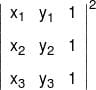= (a + b + c)(b + c – a)(c + a – b)(a + b – c)
Then the value of k is :-

Solution: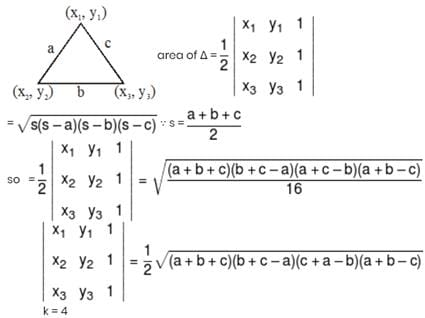*Answer can only contain numeric values
QUESTION: 24

The interior angles of a convex polygon form an arithmetic progression with a common difference of 4°. Determine the number of sides of the polygon if its largest interior angle is 172°:-

Solution: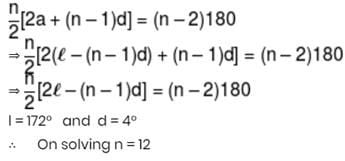*Answer can only contain numeric values
QUESTION: 25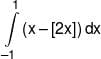equals :-

Solution: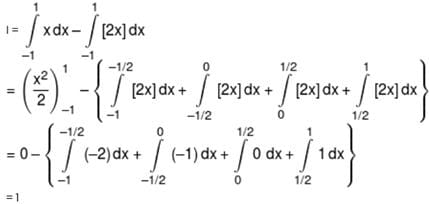Use Code STAYHOME200 and get INR 200 additional OFF Use Coupon Code